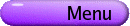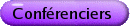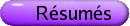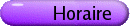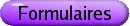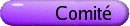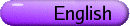Equations aux derivées partielles non linéaires
Org: Walter Craig (McMaster), Robert McCann (Toronto) et Catherine Sulem (Toronto)
[PDF]

ANDREI BIRYUK, McMaster University
Infinite energy solutions of multi-dimensional Burgers-type equations
[PDF]

We consider the Burgers-type equation

 ut + (f(u) ·Ñ) u = nDu
in Rn with L¥ (not necessary potential) initial vector field. We show that there is a unique global smooth solution, satisfying the initial condition in a weak sense.

MARINA CHUGUNOVA, McMaster University, Hamilton, ON
Diagonalization of the Hamiltonian Coupled-mode System in the Presence of Symmetry
[PDF]

We consider the Hamiltonian coupled-mode system derived in nonlinear optics, photonics, and atomic physics. Since the Dirac energy is not bounded, the standard stability analysis based on Lyapunov functionals does not work for gap solitons. Spectral stability of gap solitons depends on eigenvalues of the linearized coupled-mode equations, which are equivalent to a four-by-four Dirac system with sign-indefinite metric. In the special class of symmetric nonlinear potentials, we construct a block-diagonal representation of the linearized couple-mode equations when the spectral problem reduces to two coupled two-by-two Dirac systems.

GALIA DAFNI, Concordia University, 1455 de Maisonneuve Blvd. West, Montreal, Quebec H3G 1M8
Div-curl lemmas for local Hardy spaces and BMO
[PDF]

Nonhomogeneous versions of the div-curl lemma of Coifman, Lions, Meyer and Semmes are given in the context of local Hardy spaces (in the sense of Goldberg), on Rn and on domains. A version for BMO on domains (joint work with Chang and Sadosky) will also be discussed.

PHILIPPE GUYENNE, McMaster University, 1280 Main St. W, Hamilton, ON
Solitary wave interactions
[PDF]

We study numerically the interactions of solitary water waves for the full Euler equations. Both collisions of co- and counter-propagating solitary waves are considered. We show that the collisions are inelastic and generate small residual waves. Comparisons with KdV predictions and lab experiments will be discussed.

SLIM IBRAHIM, McMaster
On the global and ill-posedness for a 2D NLS with exponential type nonlinearity
[PDF]

In this work, we define a new criticality notion for solutions of a 2D NLS equation with exponential type nonlinearity. We prove global well-posedness in the subcritical and critical cases, while ill-posedness is shown in the supercritical case.

REINHARD ILLNER, University of Victoria
Jeffery's equation: The motion of ellipsoidal bodies in incompressible viscous flows
[PDF]

In 1922 Jeffery derived an ODE for the motion of the principal axis of a rotationally symmetric, elongated ellipsoid in a Stokes flow. The equation is

 p¢ = 1 2 (curlu0 Ùp + l(S[u0] p -(pT S[u0] p) p)
where S[u0] is the symmetric part of the velocity Jacobian, and l is a parameter depending on the geometry of the ellipsoid.

Jeffery's equation finds applications in flow problems arising in the manufacture of artifacts with immersed objects (glass, metal or plastic "sticks") to modify the elastoplastic behaviour of the product. In this talk, I will discuss some of these applications, and I will outline a new derivation of the Jeffery equation via asymptotic analysis from an exterior boundary value problem for the Navier-Stokes equations.

This is joint work with Michael Junk, Konstanz.

ROBERT JERRARD, University of Toronto
Refined Jacobian estimates and vortex dynamics for the Gross-Pitaevsky equation
[PDF]

We study dynamics of vortices in solutions of the Gross-Pitaevsky equations on 2-dimensional domains. These equations model certain superfluids, and they contain a dimensionless parameter e. Results characterizing vortex dynamics in the limit e® 0 have been known since the late '90s. Our results improve on this earlier work in several ways: they are valid for fixed small e rather than only for a sequence of solutions with e tending to zero; and they are valid for larger numbers of vortices and longer time scales than previous work. The refined Jacobian estimates mentioned in the title of the talk play a crucial technical role in the proof and are possibly of independent interest.

This is joint work with D. Spirn.

NIKY KAMRAN, McGill University
Decay of scalar waves in Kerr geometry
[PDF]

We consider the Cauchy problem for the scalar wave equation in the Kerr geometry for a rotating black hole in equilibrium, and prove that the solutions corresponding to initial data compactly supported outside the event horizon end to zero in L¥loc as t ®¥ in the Boyer-Lindquist slicing. The analysis is more difficult that in the case of the Dirac equation, owing to the presence of an ergosphere, that is a region of space-time outside the event horizon in which the Killing vector corresponding to time translations becomes space-like. This is joint work with Felix Finster, Joel Smoller and Shing-Tung Yau.

JOSE ALBERTO MONTERO, McMaster University
Stable Critical points to the Ginzburg Landau equations
[PDF]

In this talk we describe some stable solutions to the Ginzburg Landau equation that develop singularities as a small parameter goes to zero. Our main tools are the weak jacobians of Jerarrd and Soner, together with some standard gamma-convergence techniques.

VLADISLAV PANFEROV, McMaster University, Hamilton, Ontario
Regular solutions of the Boltzmann equation in 1D in space
[PDF]

I will discuss the problem of regularity of weak solutions of the nonlinear Boltzmann equation with one-dimensional (plane-wave) symmetry. By imposing certain truncations on possible configurations of collisions of particles we are able to prove that the initial-value problem has global weak solutions in L¥ provided that the initial data are essentially bounded and satisfy a mild additional condition meaning that the velocity averages obtained by free streaming remain bounded for all times. The obtained regularity is enough to guarantee uniqueness and propagation of bounds for derivatives.

FRIDOLIN TING, Lakehead University, 955 Oliver Road, Thunder Bay, ON P7B 5E1
(In)stability of pinned fundamental vortices
[PDF]

We study the (in)stability of pinned ±1 vortex solutions to the Ginzburg-Landau equations with external potentials in R2. For smooth and sufficiently small external potentials, there exists a perturbed vortex solution centered near critical points of the external potential (called pinned vortices). We show that pinned vortices centered near maxima (resp. minima) of the potential are orbitally stable (resp. unstable) w.r.t. gradient and Hamiltonian flow. This is joint work with I. M. Sigal.

AGNES TOURIN, McMaster University, HH, 1280 Main Street West, Toronto, ON L8S 4K1
A particular fully nonlinear degenerate parabolic equation arising in Finance
[PDF]

I will present a monotone and stable approximation of the fully nonlinear degenerate parabolic equation derived recently by Cheridito, Soner and Touzi from the stochastic control problem of super-replicating a contingent claim under gamma constraints. I will show some numerical results that are of practical interest. This equation is nonstandard but the theory of viscosity solutions still provides good methods for solving this type of problem in a robust manner and the convergence of the numerical scheme can be proved.

MAXIM TROKHIMTCHOUK, California - Berkeley